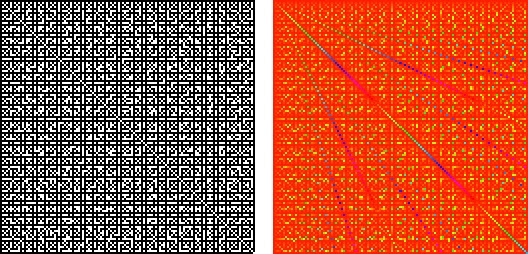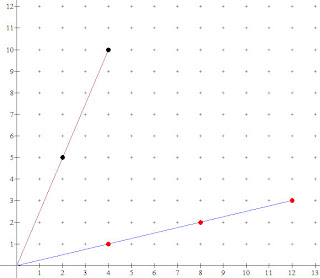## Saturday, 18 July 2009

### "It could easily be shown..." Probability and Pi and the Riemann Zeta FunctionBeware of articles that begin, "It could easily be shown..." It is like arm wrestling a two year old, if you win, so what, and if you LOSE??? Yow....
I know this, and yet, I still proceed foolishly to read them. The one currently on my mind was a "Note on Pi" by R. Chartes in the March 1904 Philosophical Magazine, my current old document of choice. It pointed out that ICEBS "that if two numbers are written down at random, the probability that they will be prime to each other is 6/pi2."
Here it is from Wolfram Mathworld:This is the reciprocal of the famous answer to the Basel problem evaluate by Euler.

The fact that the probability that two random numbers are relatively prime was equal to this value was discovered by M. Cesaro and J. J. Sylvester in the same year, 1883. Sylvester gives a proof in a footnote to a paper I found in his collected works (page 602)

Ok, yeah, that is easy, and I should have figured it out...The proof is easy to extend to the probability that three numbers are relatively prime is the reciprocal of the sum of the reciprocal of the cubes (if that seems hard to read, try to write it). Strangely, the discovery (by Sylvester) is nested in work he was doing with Farey Fractions.

The image at the top shows a pair of coordinate axes with a point (x,y) painter black if GCF(m,n)=1, and white otherwise.

I think one of the really nice things that can be done with younger students studying common factors and slope (can I say in Alg I?) is to show them that the greatest common factor of m and n is the number of lattice points on the line from (0,0) to (m,n)....[not counting (0,0)] Here is a graph of the segments to (4,10) and (12,3)